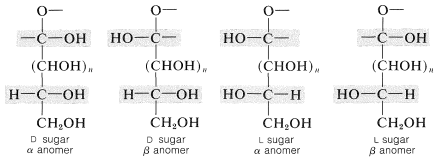# 20.4: Conventions for Indicating Ring Size and Anomer Configurations of Monosaccharides

$$\newcommand{\vecs}{\overset { \rightharpoonup} {\mathbf{#1}} }$$ $$\newcommand{\vecd}{\overset{-\!-\!\rightharpoonup}{\vphantom{a}\smash {#1}}}$$$$\newcommand{\id}{\mathrm{id}}$$ $$\newcommand{\Span}{\mathrm{span}}$$ $$\newcommand{\kernel}{\mathrm{null}\,}$$ $$\newcommand{\range}{\mathrm{range}\,}$$ $$\newcommand{\RealPart}{\mathrm{Re}}$$ $$\newcommand{\ImaginaryPart}{\mathrm{Im}}$$ $$\newcommand{\Argument}{\mathrm{Arg}}$$ $$\newcommand{\norm}{\| #1 \|}$$ $$\newcommand{\inner}{\langle #1, #2 \rangle}$$ $$\newcommand{\Span}{\mathrm{span}}$$ $$\newcommand{\id}{\mathrm{id}}$$ $$\newcommand{\Span}{\mathrm{span}}$$ $$\newcommand{\kernel}{\mathrm{null}\,}$$ $$\newcommand{\range}{\mathrm{range}\,}$$ $$\newcommand{\RealPart}{\mathrm{Re}}$$ $$\newcommand{\ImaginaryPart}{\mathrm{Im}}$$ $$\newcommand{\Argument}{\mathrm{Arg}}$$ $$\newcommand{\norm}{\| #1 \|}$$ $$\newcommand{\inner}{\langle #1, #2 \rangle}$$ $$\newcommand{\Span}{\mathrm{span}}$$

The oxide ring is six-membered in some sugars and five-membered in others, and it is helpful to use names that indicate the ring size. The five- and six-membered oxide rings bear a formal relationship to oxa-2,5-cyclohexadiene and oxa-2,4-cyclopentadiene that commonly are known as pyran and furan, respectively:

There is an important question as to which one of the two anomeric forms of a sugar should be designated as $$\alpha$$ and which one as $$\beta$$. The convention is simple; the $$\alpha$$ anomer is the one that has the same configuration of the $$\ce{OH}$$ at the anomeric carbon as the carbon which determines the configuration of the sugar itself: